# Line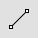Curve

Line >

<Options>

The Line command draws one line segment.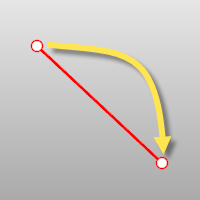#### Steps

1. Pick the start of the line.
2. Pick the end of the line.
Use object snaps to reference existing geometry.
Command-line options
##### BothSides
Draws the object on both sides of the start point, creating the object twice as long as you indicate.##### Normal

Draws a line normal to a location on a surface.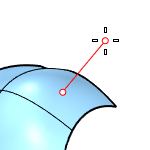A surface normal line.

#### Normal steps

1. Select a surface.
2. Pick the start of the line on the surface.
3. Pick the end of the line or type a length, and press Enter.
##### IgnoreTrims

Surface trims are ignored. When the marker misses the untrimmed surface, the no-access cursor is shown.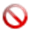No access cursor.

##### Angled

Draws the line at a specified angle from a reference line.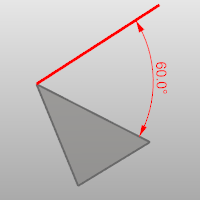#### Angled steps

1. Pick the start of a base (reference) line.
2. Pick the end of a base (reference) line.
3. Type the pivot angle, and press Enter.
4. Pick the end of the line.
##### Vertical

Draws the line vertical to the construction plane.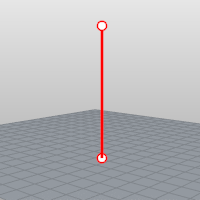#### Vertical steps

1. Pick the start of the line.
2. Pick the end of the line or type a length and press Enter.
##### FourPoint

Draws the line using two points to establish direction and two points to establish length.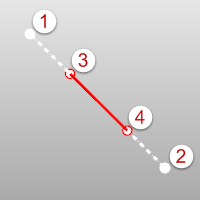#### FourPoint steps

1. Pick the start of the base line (reference location).
2. Pick the end of the base line (second reference location).
3. Pick the start of the line.
4. Pick the end of the line.
##### Bisector

The Bisector option draws the line that bisects a specified angle.#### Bisector steps

1. Pick the start of the bisector line.
2. Pick the start of the angle to bisect.
3. Pick the end of the angle to bisect.
4. Pick the end of the line or type a length, and press Enter.
##### Perpendicular

Draws the line perpendicular to or from a curve.#### Perpendicular steps

1. Pick the start of the line on a curve.
2. Pick the end of the line.
Enable the Planar mode to draw the line parallel to the current CPlane from a curve that does not lie on the CPlane.
##### Point

Allows you to pick a point that is near, but not on a curve, overriding the built-in object snap.##### FromFirstPoint

Forces the line to go through the first picked point on the curve instead of allowing the point to slide along the curve.

##### 2Curves

Restricts the line to be perpendicular to two curves.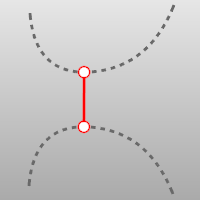##### Tangent

Draws the line tangent from a curve.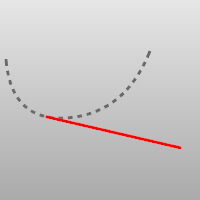#### Tangent steps

1. Pick the start of the line on a curve.
2. Pick the end of the line.
##### Point

Allows you to pick a point that is near, but not on a curve, overriding the built-in object snap.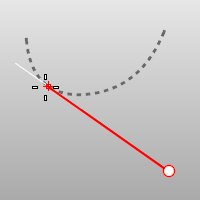##### FromFirstPoint

Forces the line to go through the first picked point on the curve instead of allowing the point to slide along the curve.

##### 2Curves

Restricts line to be tangent to two curves.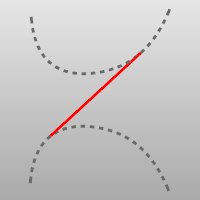##### Extension

Extends a curve with a line.#### Extension steps

1. Select a curve (line) near the end you want to extend.
2. Pick the end of the line or type a distance and press Enter.

# Lines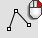Curve

Line >

Line Segments

The Lines command draw multiple adjoining line segments.

Use the Polyline command to create a single object made up of several straight or arc segments.

#### Steps

1. Pick the start of the first line segment.
2. Pick the ends of the segments, and press Enter.
Command-line options

Close

Ends the line segment string at the beginning of the series.

Undo

Reverses the last action.

# LineThroughPt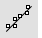The LineThroughPt command fits a line through selected points.